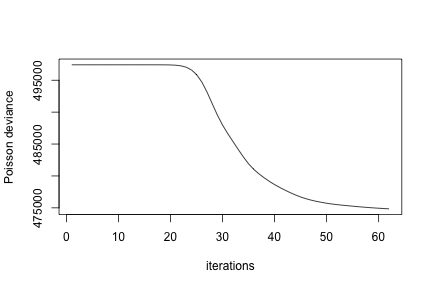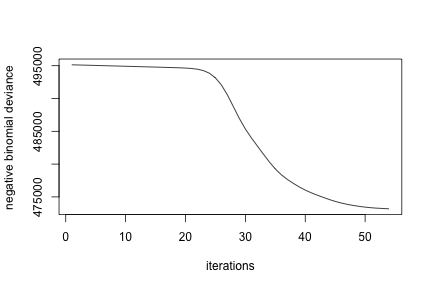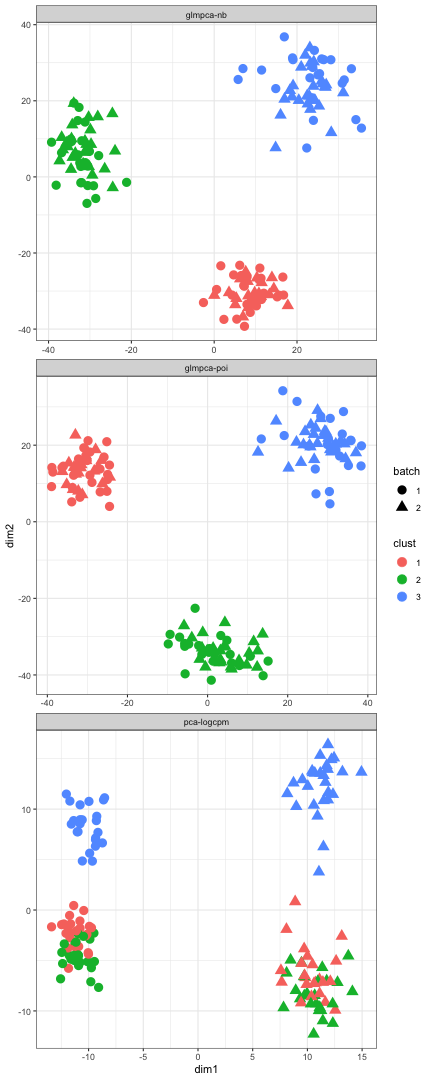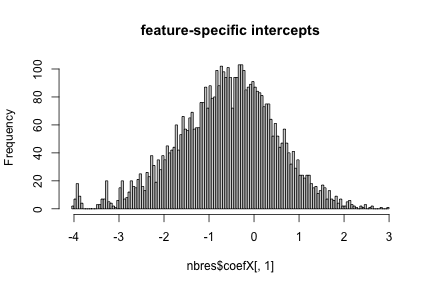library(ggplot2); theme_set(theme_bw())
require(glmpca)

Simulate some data. Thanks to Jake Yeung for providing the original inspiration for the simulation. We create three biological groups (clusters) of 50 cells each. There are 5,000 total genes and of these we set 10% to be differentially expressed across clusters. We also create two batches, one with a high total count and the other with a low total count. Each batch has an equal number of cells from the three biological clusters. A successful dimension reduction will recover the three true clusters and avoid separating cells by batch.

set.seed(202)
ngenes <- 5000 #must be divisible by 10
ngenes_informative<-ngenes*.1
ncells <- 50 #number of cells per cluster, must be divisible by 2
nclust<- 3
# simulate two batches with different depths
batch<-rep(1:2, each = nclust*ncells/2)
ncounts <- rpois(ncells*nclust, lambda = 1000*batch)
# generate profiles for 3 clusters
profiles_informative <- replicate(nclust, exp(rnorm(ngenes_informative)))
profiles_const<-matrix(ncol=nclust,rep(exp(rnorm(ngenes-ngenes_informative)),nclust))
profiles <- rbind(profiles_informative,profiles_const)
# generate cluster labels
clust <- sample(rep(1:3, each = ncells))
# generate single-cell transcriptomes
counts <- sapply(seq_along(clust), function(i){
rmultinom(1, ncounts[i], prob = profiles[,clust[i]])
})
rownames(counts) <- paste("gene", seq(nrow(counts)), sep = "_")
colnames(counts) <- paste("cell", seq(ncol(counts)), sep = "_")
# clean up rows
Y <- counts[rowSums(counts) > 0, ]
sz<-colSums(Y)
Ycpm<-1e6*t(t(Y)/sz)
Yl2<-log2(1+Ycpm)
z<-log10(sz)
pz<-1-colMeans(Y>0)
cm<-data.frame(total_counts=sz,zero_frac=pz,clust=factor(clust),batch=factor(batch))

Poisson likelihood

set.seed(202)
##    user  system elapsed
##   7.998   1.260   8.368
print(res1)
## GLM-PCA fit with 2 latent factors
## number of observations: 150
## number of features: 4989
## family: poi
## minibatch: none
## learning rate: 0.02
## final deviance: 4.749e+05
pd1<-cbind(cm,res1\$factors,dimreduce="glmpca-poi")
#check optimizer decreased deviance
plot(res1\$dev,type="l",xlab="iterations",ylab="Poisson deviance")Negative binomial likelihood

set.seed(202)
##    user  system elapsed
##  21.214   1.236  21.815
print(res2)
## GLM-PCA fit with 2 latent factors
## number of observations: 150
## number of features: 4989
## family: nb
## minibatch: none
## learning rate: 0.02
## final deviance: 4.732e+05
pd2<-cbind(cm,res2\$factors,dimreduce="glmpca-nb")
#check optimizer decreased deviance
plot(res2\$dev,type="l",xlab="iterations",ylab="negative binomial deviance")Standard PCA

#standard PCA
system.time(res3<-prcomp(log2(1+t(Ycpm)),center=TRUE,scale.=TRUE,rank.=2)) #<1 sec
##    user  system elapsed
##   0.603   0.099   0.420
pca_factors<-res3\$x
colnames(pca_factors)<-paste0("dim",1:2)
pd3<-cbind(cm,pca_factors,dimreduce="pca-logcpm")

Visualize results

pd<-rbind(pd1,pd2,pd3)
#visualize results
ggplot(pd,aes(x=dim1,y=dim2,colour=clust,shape=batch))+geom_point(size=4)+facet_wrap(~dimreduce,scales="free",nrow=3)GLM-PCA identifies the three biological clusters and removes the batch effect. The result is the same whether we use the Poisson or negative binomial likelihood (although the latter is slightly slower). Standard PCA identifies the batch effect as the primary source of variation in the data, even after normalization. Application of a clustering algorithm to the PCA dimension reduction would identify incorrect clusters.

Examining the GLM-PCA output

The glmpca function returns an S3 object (really just a list) with several components. We will examine more closely the result of the negative binomial GLM-PCA.

• Y is the data matrix whose dimension we want to reduce
• factors is a matrix whose rows match the columns (observations) of Y. It is analogous to the principal components. Each column of the factors matrix is a different latent dimension.
• coefX is a matrix of coefficients for any column-wise (observation-specific) covariates. By default, only an intercept is included. Each row of coefX corresponds to a row of Y and each column corresponds to a different covariate.
• coefZ is a matrix of coefficients for any row-wise (feature-specific) covariates. By default, no such covariates are included and this is returned as NULL.
• dev is a vector of deviance values. The length of the vector is the number of iterations it took for GLM-PCA's optimizer to converge. The deviance should generally decrease over time. If it fluctuates wildly, this often indicates numerical instability, which can be improved by increasing the penalty parameter.
• glmpca_family is an S3 object of class “glmpca_family”. This is a minor extension to the “family” object used by functions like glm and glm.nb. It is basically a list with various internal functions and parameters needed to optimize the GLM-PCA objective function. For the negative binomial case, it also contains the final estimated value of the dispersion parameter.
nbres<-res2
names(nbres)
##   "coefX"         "coefZ"         "dev"           "glmpca_family"
##   "ctl"           "offsets"       "optimizer"     "minibatch"
dim(Y)
##  4989  150
dim(nbres\$factors)
##  150   2